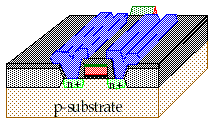Exam I

Name:

1) a) (8pts) Draw the truth table for the following circuit and indicate or briefly describe (1 - 5 words) the output state at Out for each combination of input values.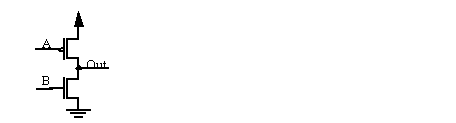b) (4pts) What is the lower bound on the number of transistors required to build a (minimal) n-input full complementary CMOS logic gate ?

c) (6 pts) Why is it necessary to use both an n-channel and a p-channel transistor to build a transmission gate ?

d) (10pts) Draw the full complementary CMOS gate that corresponds to F = (A.B)+(C.D). How many transistors do you need to implement this function if the inputs available are A, B, C and D ?

e) (6 pts) Draw the schematic diagram of a master slave flip-flop implemented using pass gates.

2a) (20 pts) Draw a schematic diagram of the magic diagram given on the next page, and presented on the overhead projector. In particular, label the input(s) and output(s) of the circuit.

b) (8 pts) Give the truth table for the circuit.

c) (4 pts) What function is/are performed at the output(s) ?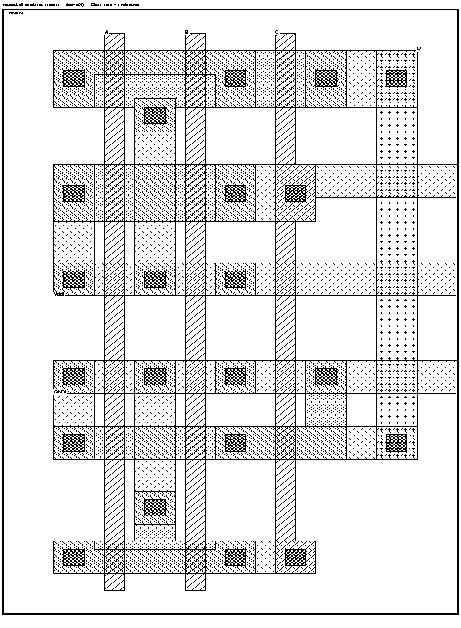3)a) (4 pts) State one version of Moore's Law.

b) (10pts) We examined the XOR gate shown below in class.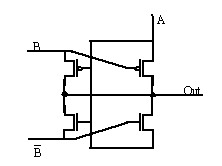Give the truth table and the schematic of a full complementary version of a 2-input XOR gate. You may want to write the boolean expression for the XOR gate and then put it into the proper form. How many transistors do we need if the inputs available are A and B ?

c) (8 pts) Briefly describe the two sources of static power dissipation in CMOS circuits. Briefly describe the two sources of dynamic power dissipation in CMOS circuits. If the power supply voltage and the process are scaled down together, what happens to speed, power and density ?

3)d) (6 pts) Magic generates layouts which are part of what domain and abstraction layer?

Magic (.mag file):

We use Magic to extract a Spice model of the circuit. What domain does the Spice representation occupy ?

Spice (.spice file):

The command `:cif write filename' generates yet another representation. What domain and abstraction layer does this representation occupy ?

Magic (.cif file):

The following boolean expression for the sum of a 1-bit adder is in what domain ?

S = A.B.C + A.B.C + A.C.B + A.B.C:

Write the structural expression for the carry out of a 1-bit adder in a high level language of your choice (e.g. C, VHDL or Verilog).

e) (6 pts) Give a one sentence description for each of the following six steps in the silicon gate process.

a)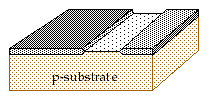b)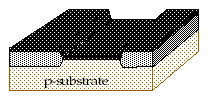c)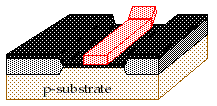d)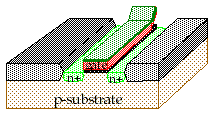e)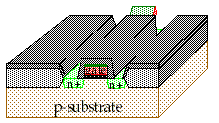f)